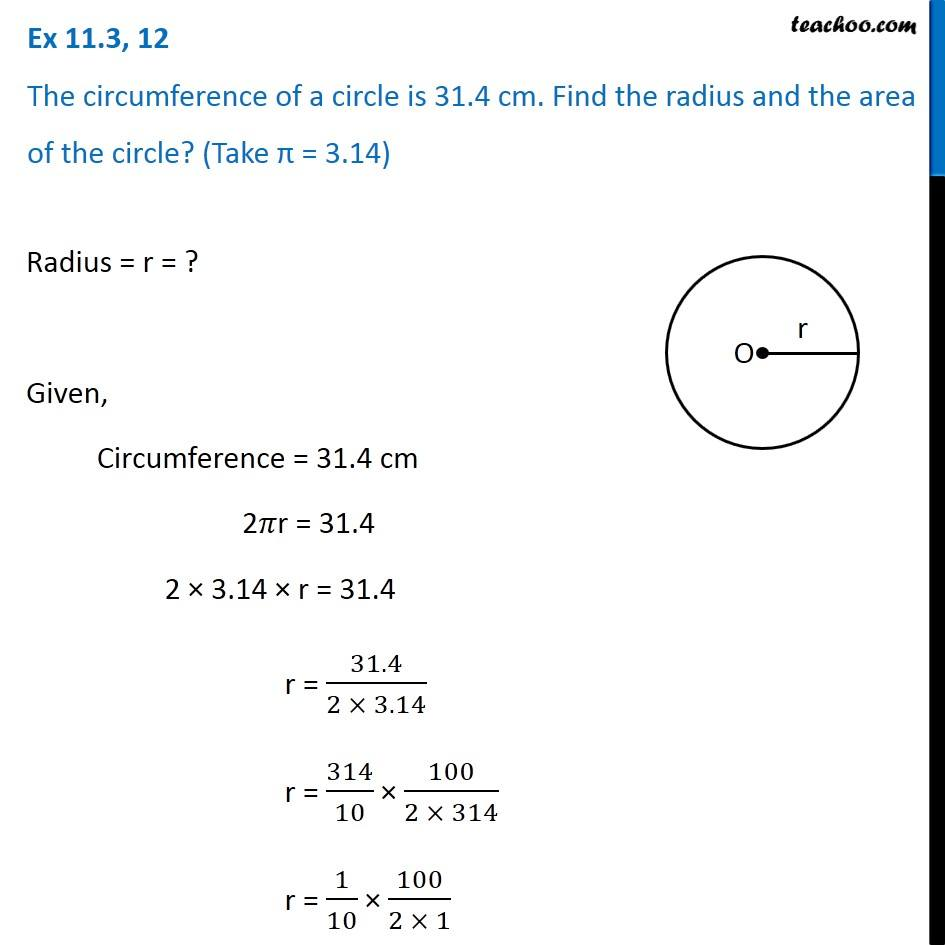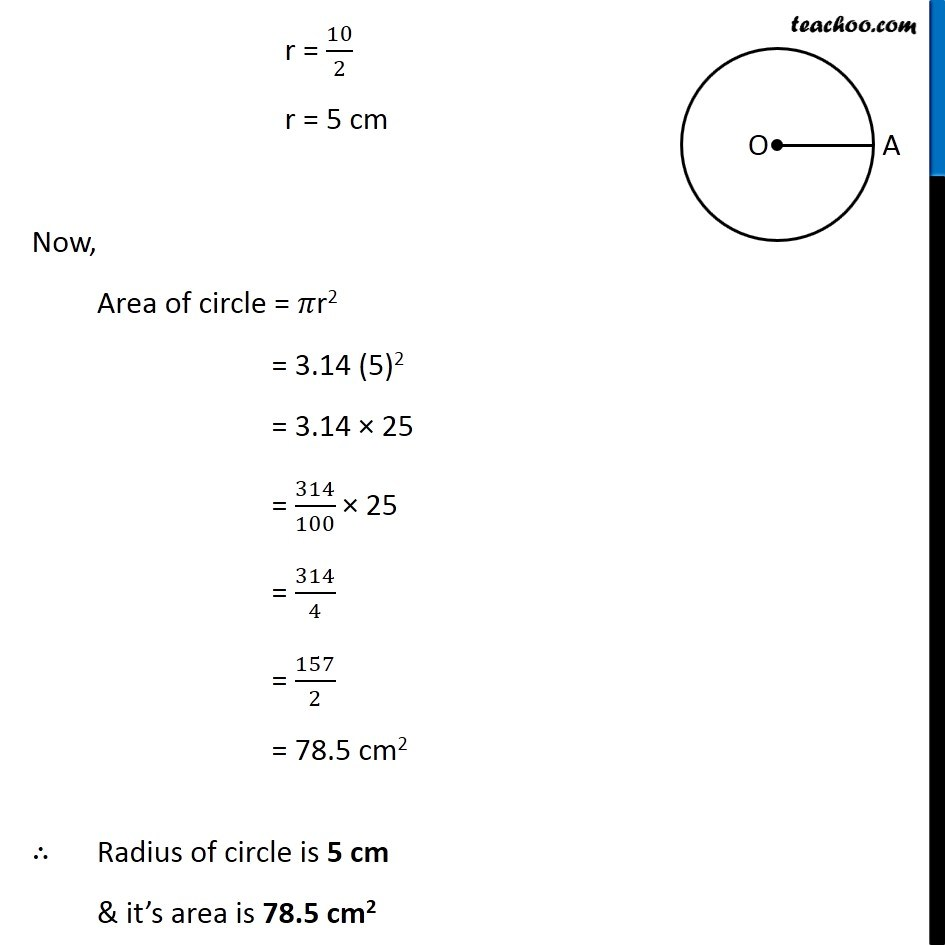Area of circle

Chapter 9 Class 7 Perimeter and Area
Concept wiseGet live Maths 1-on-1 Classs - Class 6 to 12

### Transcript

Ex 9.2, 12 The circumference of a circle is 31.4 cm. Find the radius and the area of the circle? (Take π = 3.14) Radius = r = ? Given, Circumference = 31.4 cm 2𝜋r = 31.4 2 × 3.14 × r = 31.4 r = 31.4/(2 × 3.14) r = 314/10 × 100/(2 × 314) r = 1/10 × 100/(2 × 1) r = 10/2 r = 5 cm Now, Area of circle = 𝜋r2 = 3.14 (5)2 = 3.14 × 25 = 314/100 × 25 = 314/4 = 157/2 = 78.5 cm2 ∴ Radius of circle is 5 cm & it’s area is 78.5 cm2